# Conditional logit model tutorial in Excel

2017-10-20

This tutorial will help you set up and interpret a Conditional Logit analysis in Excel using the XLSTAT statistical software.

## The conditional logit model

The conditional logit model is a statistical method similar to logistic regression.

The conditional logit model is a method mostly used in its evolved form as part of the conjoint analysis. It is nevertheless useful when to analyze a certain type of data. McFadden introduced this model in 1973. Instead of having one line per individual, there are as many lines as alternatives. Thus, it is no longer the characteristics of the individuals that are modeled but the alternatives.

If one seeks to study travel modes, we will have four travel modes (car / train / air / bus), each travel mode having its own characteristics (price, speed), but an individual can only choose one of the four modes.

As part of a conditional logit model, we have for N individuals, N*4 rows with 4 rows associated with the four choices. The binary response variable will indicate the choice of the individual with the value 1, and 0 will correspond to the options that the individual did not choose.

A column associated with the name of the individuals (with 4 lines per individual for our example) has to be selected in XLSTAT. The explanatory variables will also have N * 4 rows.

## Dataset for the conditional logit model

The example discussed below is a classic case in which one seeks to compare the travel modes proposed to go on vacation. It comes from Greene, W.H. (2003). Econometric Analysis, 5th edition. Upper Saddle River, NJ: Prentice Hall.

An Excel file containing both the data and the results can be downloaded by clicking here.

The data correspond to a sample of 210 individuals, each one having 4 possibilities (air, car, bus and train). We asked each of them the travel mode they would choose to go on vacation.

The data set has 840 rows. The first column identifies the individual, the second is the binary variable modeling the travel mode. Then there are two quantitative variables, respectively, the overall cost and the waiting time during the trip associated with each travel mode for each individual. Finally, the categorical variable associated with the transportation is in the last column (air, train, bus or car).

## Set a conditional logit model

To activate the dialog box, start XLSTAT, then select XLSTAT / XLSTAT-CJT / Conditional Logit , or click the corresponding button on the XLSTAT-CJT toolbar (see below).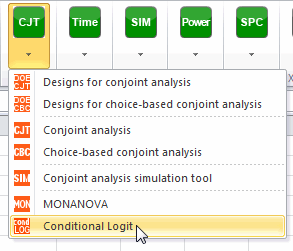Once you have clicked the button, the dialog box appears.

Select the data on the Excel sheet.

The response variable corresponds to the binary variable. The subject labels correspond to the numbers associated with the individuals (you can also have names of individuals instead). In our case there are three predictors, one qualitative - the travel mode - and two quantitative - global cost and waiting time. As we selected the labels of the variables, we must select the variable labels option.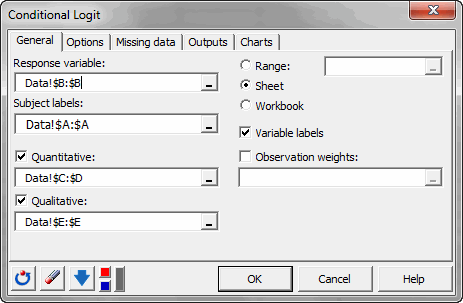Once you click the OK button, the calculations are performed and the results displayed.

## Interpret the results of a conditional logit model

The following table gives several indicators of the quality of the model (or goodness of fit). These results are similar to R² and to the analysis of variance table of linear regression and ANOVA. The most important value is the Chi-square associated with the log ratio (LR). This is equivalent to Fisher's F test in the linear model: an attempt to assess whether the variables provide a significant amount of information to explain the variability of the binary variable. In our case, as the probability is less than 0.0001, we can conclude that the variables provide a significant amount of information.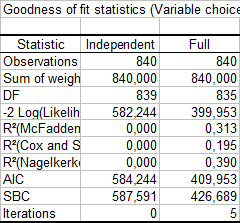These goodness of fit statistics show that our model is significantly better than the model without any predictor. The following table confirms these initial impressions: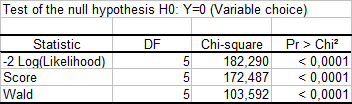The p-values are all very small and the impact of the three variables is significant in the type III analysis table.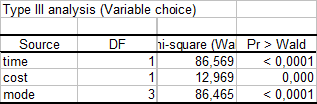Finally, the coefficients of the model show that the air is preferred and that the waiting time has a significant negative effect on the choice of travel mode.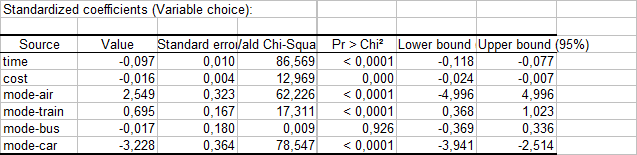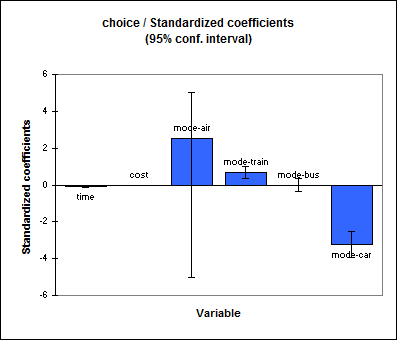The analysis of residuals may also be useful and provide other information about individuals' choices.

#### Contact our technical support team: support@xlstat.com

https://cdn.desk.com/
false
desk